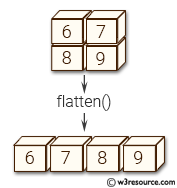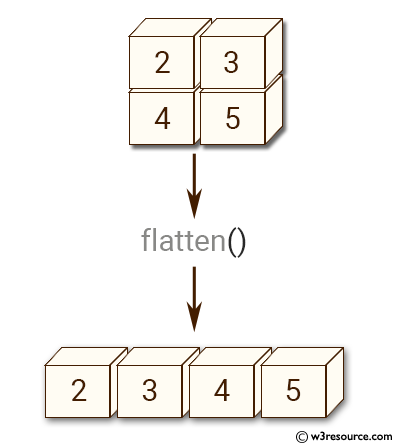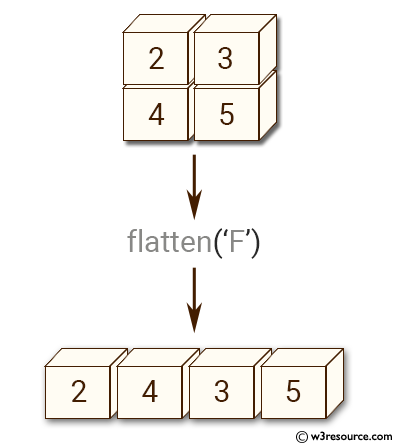# NumPy Array manipulation: ndarray.flatten() function

## numpy.ndarray.flatten() function

The flatten() function is used to get a copy of an given array collapsed into one dimension.

Syntax:

```ndarray.flatten(order='C')
```Version: 1.15.0

Parameter:

Name Description Required /
Optional
order ‘C’ means to flatten in row-major (C-style) order. ‘F’ means to flatten in column-major (Fortran- style) order. ‘A’ means to flatten in column-major order if a is Fortran contiguous in memory, row-major order otherwise. ‘K’ means to flatten a in the order the elements occur in memory. The default is ‘C’. Optional

Return value:

ndarray - A copy of the input array, flattened to one dimension.

Example-1: numpy.ndarray.flatten()

``````>>> import numpy as np
>>> y = np.array([[2,3], [4,5]])
>>> y.flatten()
array([2, 3, 4, 5])
``````

Pictorial Presentation:Example-2: numpy.ndarray.flatten()

``````>>> import numpy as np
>>> y = np.array([[2,3], [4,5]])
>>> y.flatten('F')
array([2, 4, 3, 5])
``````

Pictorial Presentation:Python - NumPy Code Editor:

Previous: ndarray.flat()
Next: Transpose-like operations moveaxis()

﻿